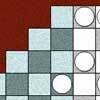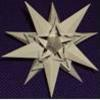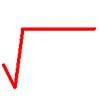# Search by Topic

Filter by: Content type:
Age range:
Challenge level:

### There are 50 results

Broad Topics > Numbers and the Number System > Powers & roots### Fit for Photocopying

##### Age 14 to 16 Challenge Level:

Explore the relationships between different paper sizes.### Power Mad!

##### Age 11 to 14 Challenge Level:

Powers of numbers behave in surprising ways. Take a look at some of these and try to explain why they are true.### Negative Power

##### Age 14 to 16 Challenge Level:

What does this number mean ? Which order of 1, 2, 3 and 4 makes the highest value ? Which makes the lowest ?### Napier's Location Arithmetic

##### Age 14 to 16 Challenge Level:

Have you seen this way of doing multiplication ?### Equal Temperament

##### Age 14 to 16 Challenge Level:

The scale on a piano does something clever : the ratio (interval) between any adjacent points on the scale is equal. If you play any note, twelve points higher will be exactly an octave on.### More Magic Potting Sheds

##### Age 11 to 14 Challenge Level:

The number of plants in Mr McGregor's magic potting shed increases overnight. He'd like to put the same number of plants in each of his gardens, planting one garden each day. How can he do it?### Magic Potting Sheds

##### Age 11 to 14 Challenge Level:

Mr McGregor has a magic potting shed. Overnight, the number of plants in it doubles. He'd like to put the same number of plants in each of three gardens, planting one garden each day. Can he do it?### Perfectly Square

##### Age 14 to 16 Challenge Level:

The sums of the squares of three related numbers is also a perfect square - can you explain why?### Guesswork

##### Age 14 to 16 Challenge Level:

Ask a friend to choose a number between 1 and 63. By identifying which of the six cards contains the number they are thinking of it is easy to tell them what the number is.### In Between

##### Age 16 to 18 Challenge Level:

Can you find the solution to this algebraic inequality?### What an Odd Fact(or)

##### Age 11 to 14 Challenge Level:

Can you show that 1^99 + 2^99 + 3^99 + 4^99 + 5^99 is divisible by 5?### Sept 03

##### Age 11 to 14 Challenge Level:

What is the last digit of the number 1 / 5^903 ?### The Root of the Problem

##### Age 14 to 18 Challenge Level:

Find the sum of this series of surds.### Number Rules - OK

##### Age 14 to 16 Challenge Level:

Can you convince me of each of the following: If a square number is multiplied by a square number the product is ALWAYS a square number...### Giants

##### Age 16 to 18 Challenge Level:

Which is the bigger, 9^10 or 10^9 ? Which is the bigger, 99^100 or 100^99 ?### Root to Poly

##### Age 14 to 16 Challenge Level:

Find the polynomial p(x) with integer coefficients such that one solution of the equation p(x)=0 is $1+\sqrt 2+\sqrt 3$.### How Many Solutions?

##### Age 16 to 18 Challenge Level:

Find all the solutions to the this equation.### Em'power'ed

##### Age 16 to 18 Challenge Level:

Find the smallest numbers a, b, and c such that: a^2 = 2b^3 = 3c^5 What can you say about other solutions to this problem?### Ab Surd Ity

##### Age 16 to 18 Challenge Level:

Find the value of sqrt(2+sqrt3)-sqrt(2-sqrt3)and then of cuberoot(2+sqrt5)+cuberoot(2-sqrt5).### Roots Near 9

##### Age 14 to 16 Short Challenge Level:

For how many integers 𝑛 is the difference between √𝑛 and 9 is less than 1?### Pocket Money

##### Age 11 to 14 Challenge Level:

Which of these pocket money systems would you rather have?### Double Trouble

##### Age 14 to 16 Challenge Level:

Simple additions can lead to intriguing results...### Function Pyramids

##### Age 16 to 18 Challenge Level:

A function pyramid is a structure where each entry in the pyramid is determined by the two entries below it. Can you figure out how the pyramid is generated?### Googol

##### Age 16 to 18 Short Challenge Level:

Find the smallest value for which a particular sequence is greater than a googol.### Archimedes Numerical Roots

##### Age 16 to 18 Challenge Level:

How did Archimedes calculate the lengths of the sides of the polygons which needed him to be able to calculate square roots?### Power Countdown

##### Age 14 to 16 Challenge Level:

In this twist on the well-known Countdown numbers game, use your knowledge of Powers and Roots to make a target.### Unusual Long Division - Square Roots Before Calculators

##### Age 14 to 16 Challenge Level:

However did we manage before calculators? Is there an efficient way to do a square root if you have to do the work yourself?### Plus or Minus

##### Age 16 to 18 Challenge Level:

Make and prove a conjecture about the value of the product of the Fibonacci numbers $F_{n+1}F_{n-1}$.### Pythagorean Fibs

##### Age 16 to 18 Challenge Level:

What have Fibonacci numbers got to do with Pythagorean triples?### Fibonacci Fashion

##### Age 16 to 18 Challenge Level:

What have Fibonacci numbers to do with solutions of the quadratic equation x^2 - x - 1 = 0 ?### St Ives

##### Age 7 to 11

As I was going to St Ives, I met a man with seven wives. Every wife had seven sacks, every sack had seven cats, every cat had seven kittens. Kittens, cats, sacks and wives, how many were going to St. . . .### Lost in Space

##### Age 14 to 16 Challenge Level:

How many ways are there to count 1 - 2 - 3 in the array of triangular numbers? What happens with larger arrays? Can you predict for any size array?### Lastly - Well

##### Age 11 to 14 Challenge Level:

What are the last two digits of 2^(2^2003)?### Largest Number

##### Age 11 to 14 Challenge Level:

What is the largest number you can make using the three digits 2, 3 and 4 in any way you like, using any operations you like? You can only use each digit once.### Enriching Experience

##### Age 14 to 16 Challenge Level:

Find the five distinct digits N, R, I, C and H in the following nomogram### Square Pair Circles

##### Age 16 to 18 Challenge Level:

Investigate the number of points with integer coordinates on circles with centres at the origin for which the square of the radius is a power of 5.### Archimedes and Numerical Roots

##### Age 14 to 16 Challenge Level:

The problem is how did Archimedes calculate the lengths of the sides of the polygons which needed him to be able to calculate square roots?### Power Crazy

##### Age 11 to 14 Challenge Level:

What can you say about the values of n that make $7^n + 3^n$ a multiple of 10? Are there other pairs of integers between 1 and 10 which have similar properties?### Deep Roots

##### Age 14 to 16 Challenge Level:

Find integer solutions to: $\sqrt{a+b\sqrt{x}} + \sqrt{c+d.\sqrt{x}}=1$### Two Many

##### Age 11 to 14 Challenge Level:

What is the least square number which commences with six two's?### Like Powers

##### Age 11 to 14 Challenge Level:

Investigate $1^n + 19^n + 20^n + 51^n + 57^n + 80^n + 82^n$ and $2^n + 12^n + 31^n + 40^n + 69^n + 71^n + 85^n$ for different values of n.### Rachel's Problem

##### Age 14 to 16 Challenge Level:

Is it true that $99^n$ has 2n digits and $999^n$ has 3n digits? Investigate!### Diggits

##### Age 11 to 14 Challenge Level:

Can you find what the last two digits of the number $4^{1999}$ are?### Consecutive Squares

##### Age 14 to 16 Challenge Level:

The squares of any 8 consecutive numbers can be arranged into two sets of four numbers with the same sum. True of false?### Staircase

##### Age 16 to 18 Challenge Level:

Solving the equation x^3 = 3 is easy but what about solving equations with a 'staircase' of powers?### Mod 7

##### Age 16 to 18 Challenge Level:

Find the remainder when 3^{2001} is divided by 7.### Rationals Between...

##### Age 14 to 16 Challenge Level:

What fractions can you find between the square roots of 65 and 67?### Rational Roots

##### Age 16 to 18 Challenge Level:

Given that a, b and c are natural numbers show that if sqrt a+sqrt b is rational then it is a natural number. Extend this to 3 variables.### The Root Cause

##### Age 16 to 18 Challenge Level:

Prove that if a is a natural number and the square root of a is rational, then it is a square number (an integer n^2 for some integer n.)### Route to Root

##### Age 16 to 18 Challenge Level:

A sequence of numbers x1, x2, x3, ... starts with x1 = 2, and, if you know any term xn, you can find the next term xn+1 using the formula: xn+1 = (xn + 3/xn)/2 . Calculate the first six terms of this. . . .